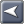﻿ Alpha, Beta, Standard Deviation
Captools/net Documentation

Alpha, Beta, Standard Deviation, etc.

 Alpha, Beta, Standard Deviation, etc.Alpha, Beta, Standard Deviation, etc.Captools/net performance reports provide you with the option of reporting "alpha", "beta", "R-squared" and "sigma" (standard deviation) performance statistical measures whenever ROI performance is also reported.  "Sharpe" and "Treynor" ratio reporting is also available.

Alpha, Beta and R-squared - These are respectively the y-intercept, slope and correlation of a best fit line through a scatter gram plot of the investment ROIs (y-axis) versus ROIs for a broad market index for the time period in question.  A positive alpha signifies that the investment is outperforming the market on a risk-adjusted basis.  A beta greater than 1.0 indicates that the investment is more volatile than the index, whereas a beta between "0" and "1.0" indicates that the investment is less risky than the market index.  A negative beta indicates the investment performance is counter-cyclical to the market.  R-squared is a measure of the statistical significance of the alpha and beta.  In general, any data with an R-squared value of less than "0.5" should be ignored.

Sigma (Standard Deviation) - This is a measure of the volatility of an investment's ROI performance, and is often considered a good indicator of the investment's risk.  It is computed as the standard deviation of a number of ROI performance observations for the security or portfolio being evaluated. This standard deviation should not be confused with other, more simplistic standard deviation measures of an investment's price.  These suffer as a measure of risk, in that they penalize upside price movements as well as downside movements.  Captools/net's "sigma", on the other hand, does not penalize consistent upward price movement.  Furthermore, it is superior to simplistic price-based "Ulcer Indices" in that those can fail to properly handle price movements due to dividend distributions.  Distributions are properly accounted for by a total return on investment measure such as is computed by Captools/net.

Sharpe Ratio - This is the ratio of an investment's performance less a risk-free rate of return, all divided by the investment's standard deviation, i.e.,:

S-Ratio = (Time-Weighted ROI - Risk-Free ROI) / Sigma

The Risk-Free Rate is the rate you specify in the Captools/net Program Preferences/Other Settings.  The one-year U.S. Treasury Bill rate is typically used for this rate.  The significance of the Sharpe ratio is that it permits you to compare investments with differing levels of risk.  In theory, the investment with the highest Sharpe Ratio is the superior investment, provided, of course, that the future mirrors the past.  The Sharpe ratio is named after Prof. William Sharpe, who earned a Nobel prize in connection with his work developing this theory and formula.

Treynor Ratio - This statistic reports the ratio of the Time Weighted ROI less the risk-free rate divided by the Beta statistic:

Treynor Ratio = (Time-Weighted ROI - Risk-Free ROI) / Beta

The Treynor ratio is similar to the Sharpe ratio in that it has the same numerator, but it uses the beta as the denominator measure of risk.

Comparison of Statistics - Alpha, Beta, Sigma and Sharpe ratio are statistically derived measures.  They are computed based upon a data sample, which is determined by the time periods between data points.  If the sample size used by two different parties in computing a statistical measure differs, the reported results will also differ.  Thus if the standard deviation for a market index is based on hourly data it will be different from that reported based on day's end or week's ending data even if the same start and end date are used.  Therefore one should not necessarily expect that statistical results reported by Captools/net will necessarily be exactly identical to that reported by another source unless you know the sampling methodology of the other source and have endeavored to duplicate it in your Captools/net data.

For a more thorough treatment of these statistical performance measures, we suggest you consult a university level financial textbook, or an advanced book on investing.